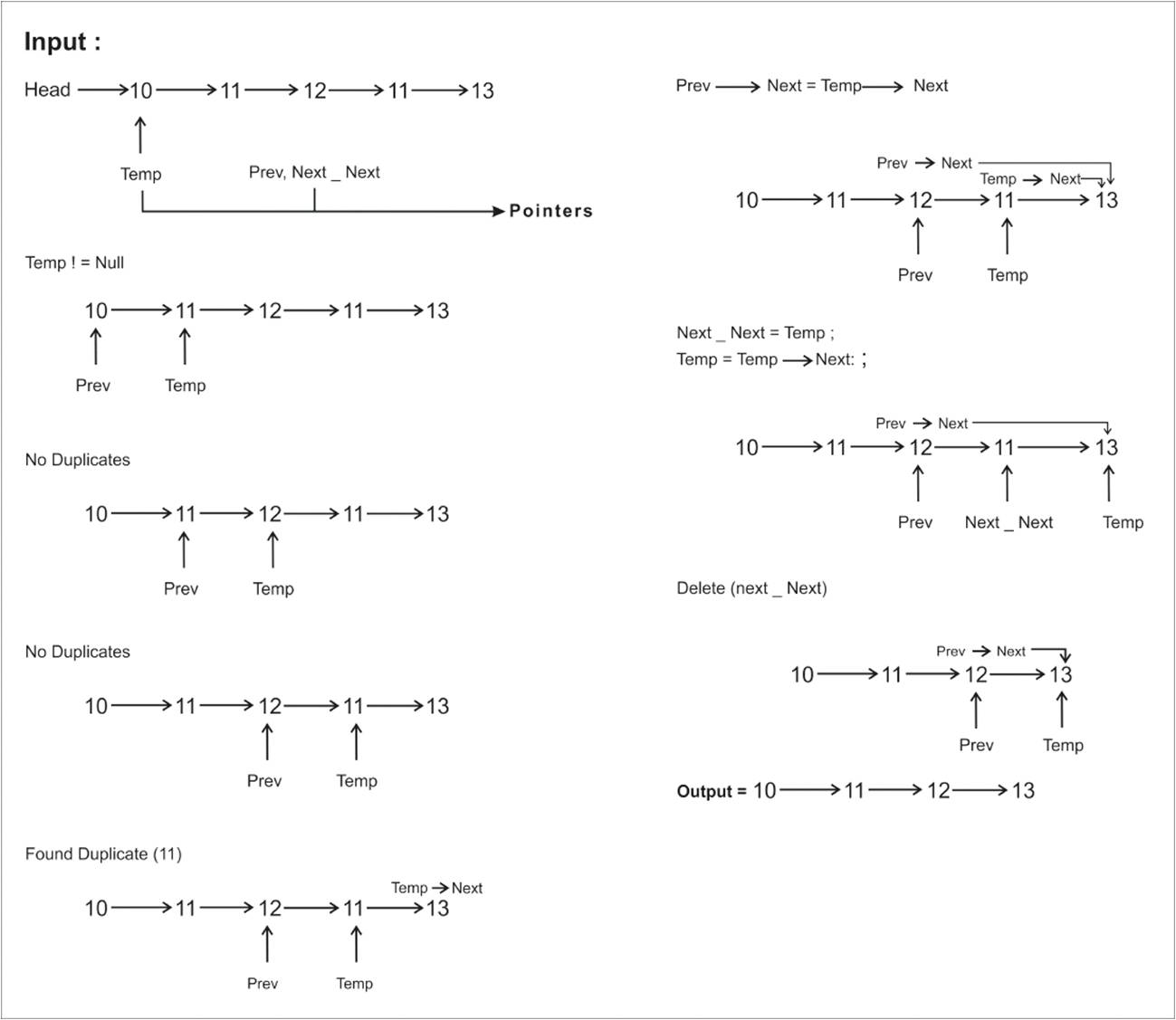Remove all duplicates in an unsorted linked list

Given an unsorted linked list, write a program to remove all the duplicate elements in the unsorted linked list

Example

Input : 10 ->12 ->11 ->11 ->12 ->11 ->10
Output : 10 ->12 ->11

This is one of the method which uses hashing to remove the duplicates in an unsorted linked list

Time Complexity: O(n),

where n is the number of nodes in the linked list, and assuming that hash table access time is O(1)

Implementation of Removing Duplicates in a unsorted listAlgorithm

1. Create three pointers (ex: temp, next_next, prev), where temp pointer is to traverse the linked list,  prev pointer is to point the node behind the temp(prev always follows temp) and the next_next is to store the node that should be deleted.

2. Till the end of the lined list, if the data is duplicate
a. point the prev's next to the temp's next ie, prev->next= temp->next
b. we need to delete the data in temp, so store it in next_next ie, next_next = temp
c. go to the next node ie, temp = temp->next

3. if the data is not duplicate
a. point the previous node to the current node ie, prev = temp
b. move the current node to current next node ie, temp = temp->next

C++ Program

``````#include<bits/stdc++.h>
using namespace std;

struct LL
{
int data;
struct LL *next;
};

map<int ,int>M;

{
struct LL *temp =new LL;
temp->data = num;
}

{
while(temp!=NULL)
{
if(temp->next!=NULL)
cout<<temp->data<<" ->";
else
cout<<temp->data;

temp=temp->next; //move to next node
}
//O(number of nodes)
cout<<endl;
}

{
struct LL *temp = *head, *prev=NULL, *next_next; //prev to store previous node and next_next to store next node of the current node

while(temp != NULL)
{
if(M[temp->data]) // if the value if already found
{
prev->next = temp->next;
next_next=temp;
temp=temp->next;
delete(next_next);
continue;
}

prev = temp;
M[temp->data] = 1; //intialize with count 1
}
}

int main()
{

cout<<"Current List is :-\n";